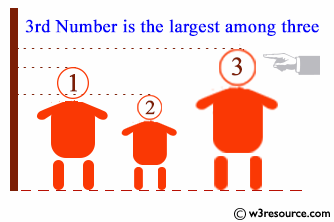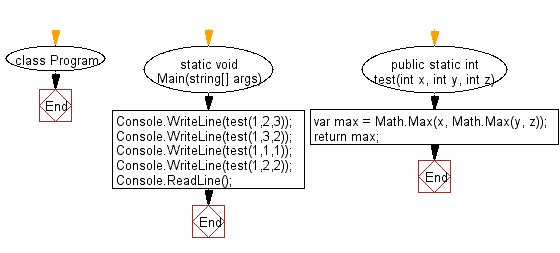﻿ C Sharp - Find the largest number among three integers# C# Sharp Basic Algorithm Exercises: Check the largest number among three given integers

## C# Sharp Basic Algorithm: Exercise-18 with Solution

Write a C# Sharp program to check the largest number among three given integers.

Pictorial Presentation:Sample Solution:

C# Sharp Code:

``````using System;

namespace exercises
{
class Program
{
static void Main(string[] args)
{
Console.WriteLine(test(1,2,3));
Console.WriteLine(test(1,3,2));
Console.WriteLine(test(1,1,1));
Console.WriteLine(test(1,2,2));
}

public static int test(int x, int y, int z)
{
var max = Math.Max(x, Math.Max(y, z));
return max;
}
}
}
```
```

Sample Output:

```3
3
1
2```

Flowchart:C# Sharp Code Editor:

Improve this sample solution and post your code through Disqus

What is the difficulty level of this exercise?

Test your Programming skills with w3resource's quiz.

﻿